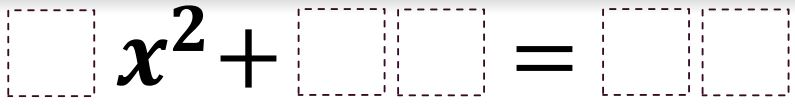# Imaginary Solutions to a Quadratic Equation

Directions: Using the digits 1 to 9 at most one time each, place a digit in each box to create a quadratic equation with an imaginary solution of the form ±𝒃𝒊 where 𝒃 is a whole number.### Hint

What needs to happen for the solution to be imaginary? How can you guarantee that 𝒃 will be a whole number?